# Maharashtra Board Class 8 Geography Solutions Chapter 1 Local Time and Standard Time

Balbharti Maharashtra State Board Class 8 Geography Solutions Chapter 1 Local Time and Standard Time Notes, Textbook Exercise Important Questions and Answers.

## Maharashtra State Board Class 8 Geography Solutions Chapter 1 Local Time and Standard Time

Class 8 Geography Chapter 1 Local Time and Standard Time Textbook Questions and Answers

1. Complete the sentence by selecting the correct option:

Question a.
The earth requires 24 hours for one rotation. In one hour, ……………. .
(a) 5 longitudes will face the sun
(b) 10 longitudes will face the sun
(c) 15 longitudes will face the sun
(d) 20 longitudes will face the sun
(c) 15 longitudes will face the sun

Question b.
To calculate the difference between the local times of any two places on the earth, …………. .
(a) the noon time at both the places should be known
(b) the difference in degrees of their longitudes should be known
(c) the difference in standard times of both the places should be known
(d) changes need to be made according to International Date Line.
(b) the difference in degrees of their longitudes should be known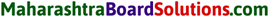Question c.
The difference between the local time of any two consecutive longitudes is …………… .
(a) 15 minutes
(b) 04 minutes
(c) 30 minutes
(d) 60 minutes
(b) 04 minutes

2. Give geographical reasons for the following:

Question a.
The local time is decided by the noontime.
1. When at a particular place, the sun reaches the maximum height in the sky it is assumed that almost half of the daytime is over and this time is considered 12 noon.

2. The time of a particular place as decided by the overhead position of the sun in the sky is considered as the local time of that place.

3. During the rotation of the earth, when a particular longitude comes exactly in front of the sun, it is considered as noontime (12 noon) on that longitude. This noontime is considered as local time of that longitude.

In this way, the local time is decided by the noontime.Question b.
The local time at Greenwich is considered to be the International Standard Time.

1. The International Standard Time has been decided according to 0° longitude.
2. 0° longitude passes through Greenwich, England.
3. For an international coordination, it is essential to bring compatibility between the standard times of various countries in the world.

For this purpose, the local time at Greenwich is considered to be the International Standard Time.

Question c.
The standard time of India has been decided by the local time at 82°30′ E longitude.

1. With respect to the longitudinal extent, 82°30’E longitude passes through the middle of India.
2. The difference between the local time at 82°30’E longitude and the local time at the extreme east and west longitude passing through India is not more than one hour.

Therefore, the standard time of India has been decided by the local time at 82°30’E longitude.

Question d.
Canada has 6 different standard times.

1. The longitudinal extent of Canada is between 52°37′ W and 141° W.
2. Thus, the difference between the extreme east and west longitude passing through Canada is of 88 degrees.
3. The difference between the local time at extreme east and west longitude passing through Canada is of 352 minutes i.e. 5 hours and 52 minutes.

Therefore, it is not practically helpful to consider single standard time in Canada. Therefore, for synchronizing the routine activities in the country, Canada has 6 different standard times.Question a.
If it is 12 noon at 60° E longitude, then explain what would be the time at 30° W longitude.
1. The difference between 60° E longitude and 30° W longitude will be of 90 degrees. (The difference of 60 degrees between 0° and 60° E + the difference of 30 degrees between 0° and 30° W = 90 degrees.)

2. Difference in local time = 90 × 4
= 360 minutes.
= 360 minutes ÷ 60 minutes = 6 hours.
3. Longitudes lying to the east of any longitude are ahead of the time of that longitude while those lying to the west are behind.
4. Therefore, if it is 12 noon at 60° E longitude, then it would be 6 a.m. at 30° W longitude, (behind by 6 hours)

Question b.
How is the standard time of a place determined?
1. The local time at the longitude passing through the middle of a country/ place is generally considered as a standard time of that country/place.

2. If the difference between the local time at the extreme east and west longitude passing through a country is less than one or two hours, one standard time is considered for a country. Thus, there exists only one standard time in a country having comparatively less longitudinal (east-west) extent.

3. If the difference between the local time at the extreme east and west longitude passing through a country is more than one or two hours, more than one standard time zones are considered for a country. Thus, there exists more than one standard time zones in a country having comparatively more longitudinal (east-west) extent.

Question c.
A football match being played at Sao Paulo, Brazil started in India at 6 a.m. IST. Explain what would be the local time at Sao Paulo?
1. Statement: For any longitude lying to the west of particular longitude, the local time decreases by 4 minutes for every longitude. (Sao Paulo is located to the west of India)

2. The difference between the longitudes of Sao Paulo and India = 127°30’.

3. Difference in local time = 127.5 × 4
= 510 minutes.
= 510 minutes ÷ 60 minutes
= 8 hours 30 minutes.

4. Thus, if it is 6 a.m. at India, it would be 9.30 p.m. of the previous day at Sao Paulo. Therefore, if a football match being played at Sao Paulo, Brazil started in India at 6 a.m. 1ST, the local time at Sao Paulo would be 9.30 p.m. of previous day.4. Complete the following table:

If it is 10 pm on 21st June at Prime Meridian, write the dates and time at A, B and C in the following table:
(Note: The answer is given directly.)

Question 1.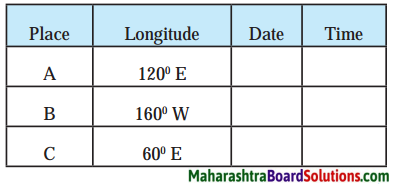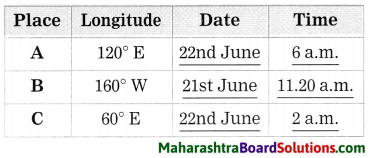5. Write the situation of place A shown in these diagrams in the boxes below them:

Question 1.
Write the situation of place A shown in these diagrams in the boxes below them
(i) Sunrise
(ii) Midnight
(iii) Noon
(iv) Sunset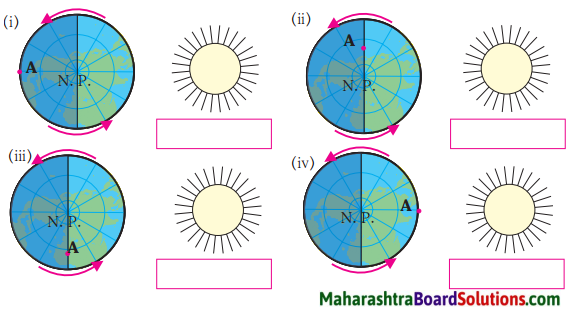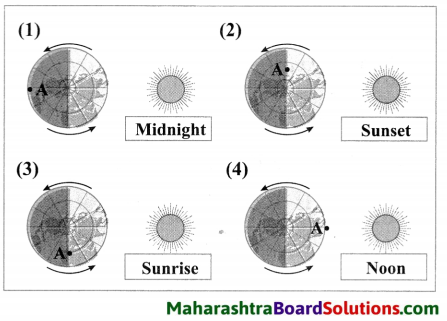Let’s recall:

Question 1.
Why does the duration of day and night keep changing?
The duration of day and night keeps changing as the earth is tilted at an angle of 23.5 degrees.Question 2.
How many longitudes can be drawn on a world map keeping an interval of 1° each?
360 longitudes can be drawn on a world map keeping an interval of 10 each.

Question 3.
The apparent movement of the sun from east to west is a result of what?
The apparent movement of the sun from east to west is a result of the rotation of the earth from west to east.

Question 4.
What is the direction of the rotation of the earth?
The direction of the rotation of the earth is from west to east.

Question 5.
While the earth rotates, how many longitudes face the sun daily?
While the earth rotates, 360 longitudes face the sun dally.

Question 6.
At which longitude does the date change?
The date changes at 1800 longitude.Question 7.
How was the time measured in olden days?
In olden days, the time was measured with the help of the natural events of sunrise and sunset and the instruments like Ghatikapaatra, sand timer, etc.

Question 8.
In present tunes, what are the instruments used for time measurement?
In present times, the instruments like watches, calendars, etc. are used for time measurement.Activity:

Question 1.
Look for the actual granny’s clock in Shri Acharya Atre’s poem: “Aajiche Ghadyal” (Granny’s clock). Look for this poem on the internet or in reference book.

Question 2.
Find out the velocity of the earth’s rotation in km/hour.

Class 8 Geography Chapter 1 Local Time and Standard Time Additional Important Questions and Answers

Complete the sentence by selecting the correct option:

Question a.
There are …………. time zones in the world.
(a) 360
(b) 24
(c) 100
(d) 365
(b) 24Question b.
Indian Standard Time is …………..
(a) ahead of Greenwich Mean Time by 5 hours 30 minutes
(b) ahead of Greenwich Mean Time by 3 hours 50 minutes
(c) behind Greenwich Mean Time by 5 hours 30 minutes
(d) behind Greenwich Mean Time by 3 hours 50 minutes
(a) ahead of Greenwich Mean Time by 5 hours 30 minutes

Examine the following statements and correct the incorrect ones:

Question 1.
Mumbai is located at 73° E longitude.
Correct.

Question 2.
In India, three standard times exist.
Incorrect.
Correct statement: In India, one standard time exists.

Question 3.
If the difference between two longitudes is of 20 degrees, the difference in their local times will be of four hours.
Incorrect.
Correct statement: If the difference between two longitudes is of 20 degrees, the difference in their local times will be of 1 hour and 20 minutes.

Answer the following questions in one sentence each:

Question 1.
Question a.
What is the maximum number of local times that can there be in the world?
The maximum number of local times that can be there in the world is 360.Question b.
How many longitudes pass the sun in one hour?
15 longitudes pass the sun in one hour.

Question 2.
How much time does the earth take to complete one rotation?
The earth takes nearly 24 hours to complete one rotation.

Question 3.
What is meant by the local time of a place?
When the sun is directly overhead at a particular place, it is considered as noon at that place and that time is considered as a local time of that place.

Solve the following:

1. Try this:

Question A.
Mashad, a town in Iran, is located on the 60° E longitude. When it is 12 noon at Greenwich, calculate the local time of Mashad town.
1. Statement: As we move towards the east of the Prime Meridian, the local time increases by four minutes for every longitude. (Mashad is located to the east of Greenwich.)

2. The difference between the longitudes of Greenwich and Mashad = 60°.

3. Difference in local time
= 60 × 4
= 240 minutes.
= 240 minutes ÷ 60 minutes
= 4 hours. ‘

4. Therefore, when it is noon at Greenwich, it would be 4 p.m. at Mashad. (Ahead of Greenwich Mean Time by 4 hours.)Question B.
Manaus city in Brazil is located on 60° W longitude. What would be the local time at Manaus when it is 12 noon at Greenwich?
1. Statement: As we move towards the west of the Prime Meridian, the local time decrease by four minutes for every longitude. (Manaus is located to the west of Greenwich.)

2. The difference between the longitudes of Greenwich and Manaus = 60°.

3. Difference in local time = 60 × 4
= 240 minutes.
= 240 minutes ÷ 60 minutes
= 4 hours.

4. Therefore, when it is noon at Greenwich, it would be 8 a.m. at Manaus. (Behind Greenwich Mean Time by 4 hours.)

2. Can you tell?

Question A.
Mumbai is located at 73° E longitude. Kolkata is located at 88° E longitude. Find the difference between the longitudes of these two cities.
Mumbai is located at 73° E longitude. Kolkata is located at 88° E longitude. Therefore, the difference between the longitudes of these two cities would be of (88 – 73 = 15) 15°.

Question B.
If the local time at Mumbai is 3 p.m. then what would be the local time at Kolkata?
1. Statement: For any longitude lying to the east of particular longitude, the local time increases by 4 minutes for every longitude.

2. The difference between the longitudes of Mumbai and Kolkata = 15°.

3. Difference in local time = 15 × 4
= 60 minutes.
= 60 minutes -r- 60 minutes = 1 hour.

4. Kolkata is located to the east of Mumbai. Therefore, if it is 3 p.m. at Mumbai, then it would be 4 p.m. at Kolkata. (ahead by 1 hour)

Answer the following questions in brief:

Question a.
Give brief information about the Indian Standard Time.
1. The Indian Standard Time (IST) is calculated on the basis of 82°30’E longitude, passing through Mirzapur, near Allahabad in Uttar Pradesh.

2. With respect to longitudinal extent, 82°30′ E longitude passes through the middle of India.

3. When the sun is directly overhead on this longitude, it is considered that it is 12 noon at every place in India. Thus, the local time at 82°30’E longitude is considered as the Standard time of India.

4. The difference between the local time at 82°30′ E longitude and the local time at the extreme east and west longitude passing through India is not more than one hour.Question b.
Give brief information about Universal Standard Time.
1. As the standard time is essential for synchronizing routine activities in a country with comparatively more j longitudinal extent, the universal standard i time is essential for an international coordinating between the countries in the world.

2. For this purpose, the local time at Greenwich (Greenwich Mean Time) in England is considered to be the International/Universal Standard Time (UST).

3. With reference to GMT, the differences in standard times of various countries in the world are calculated.

4. The Indian Standard Time is ahead of GMT by 5 hours and 30 minutes. For example, if it is 12 noon at Greenwich, then it would be 5.30 p.m. in India.

Give geographical reasons for the following:

Question a.
In the countries with larger area, it is convenient to use one standard time instead of more than one local times.
1. In the countries with larger area, much difference between the local times at extreme east and west longitude passing through the countries is found.

2. In such countries, if more than one local times are followed, it could lead to lots of confusion and chaos.

3. For example, if more than one local times are followed, it will become difficult to synchronize the timetable of railways, airways, etc. Therefore, in countries with comparatively larger area, it is convenient to use one standard time instead of more than one local times.

Study the following map/ figure/graph and answer the following questions:

1. Can you tell?
Study the figure 1.3 given on page 4 of the textbook and answer the following questions:

Question 1.
Between which longitudes does the region experience daytime?
The region shown between 90° W and 90° E in the figure experiences daytime.

Question 2.
Which longitudes experience noon and the midnight respectively?
0° longitude and 180° longitude experience noon and the midnight respectively.Question 3.
Edward from New Orleans is on which longitude?
Edward from New Orleans is on 90° W longitude.

Question 4.
What is the time at Accra city?
It is 12 noon at Accra city.

Question 5.
At the same time, what is Sharad from Patna and Yakaito from Japan doing? What time is it in these cities?
At the same time, Sharad is returning home from school and Yakaito from Japan is preparing for the night’s sleep. At the same time, it is 5.30 p.m. at Patna and 9.20 p.m. at Japan.

Question 6.
Select any one longitude. Calculate the local time of the longitudes lying 1° to the west and east of this longitude.

1. The selected longitude: 60° E. The local time of the selected longitude is 4 p.m.
2. The local time of the longitude lying 1° to the west of the selected longitude (59° E) will be 3.56 p.m.
3. The local time of the longitude lying 1° to the east of the selected longitude (61° E) will be 4.04 p.m.

2. Can you tell?

Study the figure 1.4 given on page 6 of the textbook and answer the following questions:

Question 1.
Considering the longitudinal extent of India, how many longitudes with a difference of 1° can be drawn on a map?
Considering the longitudinal extent of India, 29 longitudes with a difference of 1° can be drawn on a map.Question 2.
By how many minutes do two consecutive longitudes differ?
Two consecutive longitudes differ by 4 minutes.

Question 3.
What is the value of degrees of longitude at Mirzapur?
The value of longitude at Mirzapur is 82°30′.

Question 4.
If it is 8 a.m. at 82°30′ E, what would be the time in their clocks at the following places: Jammu, Madurai, Jaisalmer, Guwahati.
If it is 8 a.m. at 82°30′ E, it would be 8 a.m. in their clocks at Jammu, Madurai, Jaisalmer, Guwahati.
(Note: In India, the time at 82°30′ E is considered as standard time of India and therefore the same time will be considered everywhere in India.)

Question 5.
Though the distance between them is more why doesn’t the standard time differ in these places?
In India, the time at 82°30′ E is considered as standard time of India and therefore the same time will be considered everywhere in India. Therefore, though the distance between them is more, the standard time doesn’t differ in these places.Thought-Provoking Questions:

Question 1.
At the poles, sunrise occurs on one equinox and sunsets on the next equinox. If you happen to be at any of the poles during this time, then what would be the route of the sun in the daytime?
At the poles, sun rises on one equinox and sets on the next equinox. If we happen to be at any of the poles during this time, then the following would be the route of the sun in the daytime:
(A) North Pole:
1. The sun will rise at the North Pole approximately on March 21.

2. Approximately, from March 21 to June 21, the sun will rise higher in the sky with each advancing day. It will reach the maximum height approximately on June 21. Approximately, from June 21 to September 21, the sun will start sinking towards the horizon in the sky with each advancing day.

3. The sun will set at the North Pole approximately on September 21.

(B) South Pole:
1. The sun will rise at the South Pole approximately on September 21.

2. Approximately, from September 21 to December 21, the sun will rise higher in the sky with each advancing day. It will reach the maximum height approximately on December 21.

3. Approximately, from December 21 to March 21, the sun will start sinking towards the horizon in the sky with each advancing day.

4. The sun will set at the South Pole approximately on March 21.Question 2.
On which day, would the sun appear at the highest point in the sky?

1. On the North Pole, approximately on June 21, the sun would appear at the highest point in the sky.
2. On the South Pole, approximately on December 21, the sun would appear at the highest point in the sky.

Question 1.
Tick ✓ the time in the boxes which you can tell without using clock.
(Note: The answer is given directly.)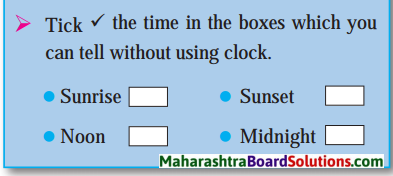3. Give it a try.

Question 1.
If it is 8 a.m. in India, what is the time in Greenwich?
If it is 8 a.m. in India, it would be 2.30 a.m. in Greenwich.Question 2.
When it is 2 p.m. in India, in which countries would it be 2 p.m. exactly?
When it is 2 p.m. in India, it would be 2 p.m. exactly in Sri Lanka.

Question 3.
When it is 9 a.m. in India, what would be the time at 82°30′ W longitude?
When it is 9 a.m. in India, it would be 10 p.m. of the previous day at 82°30′ W longitude.

Question 4.
What would be the time at Prime Meridian when a new day starts at 180° longitude?
It would be 12 noon at Prime Meridian when a new day starts at 180° longitude.

Question 1.
In which of the following countries, does only one standard time exist? (Mexico, Sri Lanka, New Zealand, China)
From the given countries, only one time exists in Sri Lanka, New Zealand and China.Question 2.
Why does a country having a large latitudinal extent have only one standard time?